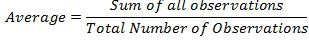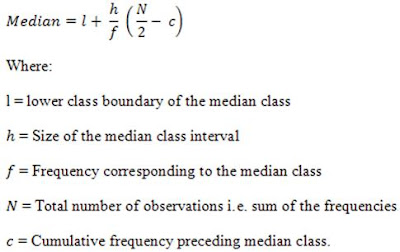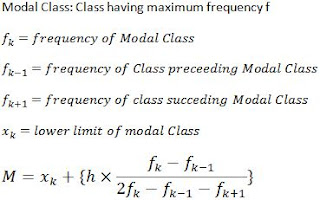## Revision Notes for Ch 14 Statistics Class 10th Mathematics

Concept of Mean, Median and Mode

• Average or mean is the certain value representative of the whole data and signifying its characteristics is called average of the data. It is also known as mean.

• The average is given as• Median of data is central data, obtained when data is arranged in ascending order. Mode is the data, having maximum frequency.

• Mode is data having maximum Frequency.

Grouped Data

• Presenting a large number of data, we need to group data for certain range, and get the frequency of that group.

Marks Obtained Frequency
0-4 3
4-9 1
10-14 7
15-19 3
20-24 6

Here, frequency of class Size is 0-4 is 3. It means 3 students has obtained marks in the range of 0-4.

• Above is the grouped the data, having range of 4 marks, i.e 0-4, 4-9.These groupings are called ‘classes’ or ‘class-intervals’, and their size is called the class-size or class width.

• Class width is chosen according convenience.

Consider the following Grouped Data

Class Frequency
0-10 2
10-20 4
20-30 5
30-40 3

In class size, if there is 10 as one of the observation, it is not represented in 0-10. It is represented in 10-20.  Similarly, If 20 is one of the data; it is included in 20-30. Not in 10-20. So, upper limit is included, and lower limit is excluded.

Inclusive Grouped Data

In this, both upper limit and lower limit is included.

Consider the following Grouped Data

That is in class 0-9, both 9 is also included.

ClassFrequency
0-93
10-195
20-294
30-393

That is in class 0-9, both 9 is also included.

Direct Method for Finding MeanAssumed Mean MethodMean of Inclusive Data

• To find mean of inclusive series, it is first needed to be converted into exclusive series.

• It is done, by subtracting 0.5 from upper and adding 0.5 to  lower limit. After that, you can calculate mean by whatever method you like, or by the method which is mentioned in question.

• The class size will be 1 more for exclusive series for corresponding inclusive series.

Note: After converting Inclusive series in exclusive series, we can find mean, median and mode of the given data.

Example:

 Class 15-19 20-24 25-29 30-34 35-39 40-44 45-49 Frequency 3 13 21 15 5 4 2

So, here we have inclusive series, We will convert it into exclusive like below

 Class 15.5-19.5 20.5-24.5 25.5-29.5 30.5-34.5 35.5-39.5 40.5-44.5 45.5-49.5 Frequency 3 13 21 15 5 4 2

Cumulative Frequency

• Cumulative frequency is the running total of all frequencies.

Consider the following Data

• The following data represent the marks obtained by 100 students in science test.

 Marks Frequency (Number of Students) Cumulative Frequency 0-10 5 5 10-20 20 25 20-30 45 70 30-40 20 90 40-50 10 100

As you can see that, cumulative frequency is the sum of all frequencies of all class size, till that class.

What Cumulative frequency signifies?

 Marks less than Number of students 10 5 20 25 30 70 40 90 50 100

So, by using cumulative frequency, we get that, how many students scored less than a particular mark. Similarly, we can represent the above data, in following form.

Less Than

 Marks less than Number of students 10 5 20 25 30 70 40 90 50 100

More Than

 Marks less than Number of students 0 100 10 95 20 75 30 30 40 10 50 0

MedianModeMode= 3(median)-2(mean)

Cumulative Frequency Curve (Ogive)

Tabulate the ‘less than’ and ‘more than’ cumulative series, as mentioned earlier.

• On a graph paper, we mark the upper class limits along X axis and corresponding cumulative frequencies along y axis.

• Take a point A(0,N/2) on the y axis and draw AP II X-axis, cutting the above curve at a point P. Draw PM perpendicular to x-axis.

• We can draw it for both greater than and lower than series.

• The point as much both curve intersect is median.

• The curve obtained by less than series is known as less than ogive. The curve obtained by more than series is known as more than Ogive.

Q. Draw less than and more than Ogive of the following data, and find the median.

 Capital (In lakh of Rs) Number of Companies 0-10 2 10-20 3 20-30 7 30-40 11 40-50 15 50-60 7 60-70 2 70-80 3

Solution:

First we will find sum of all frequencies.
Now, we will draw a more than series table of the above data.

 Capital (In lakh of Rs) Number of Companies 0 50 10 48 20 45 30 38 40 27 50 12 60 5 70 3 80 0

Now, we will draw less.

 Capital (In lakh of Rs) Number of Companies 0 0 10 2 20 5 30 12 40 23 50 38 60 45 70 47 80 50

Now, we will plot Graphs for both, one by one.